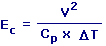Eckert Number Calculator Enter value, select unit and click on calculate. Result will be displayed.Ec = Eckert Number V = Velocity cp = Specific Heat DT = Temperature Difference
 Enter your values: Velocity (V): Meter / Second Foot / Second Centimeter / Hour Centimeter / Second Foot / Day Inch / Hour Inch / Second Kilometer / Second Knot Meter / Day Mile / Hour Mile / Second Millimeter / Second Yard / Second Specific Heat (cp): Joule / Kilogram - Kelvin Calorie / Gram - Celsius Temperature Difference (DT): Kelvin Celsius Fahrenheit Result: Eckert Number (Ec):

 Web www.calculatoredge.com
 The Eckert number is a dimensionless number used in flow calculations. It is named after professor Ernst R. G. Eckert.

It gives the relationship between a flow's kinetic energy and enthalpy, and is used to characterize dissipation.

Above equation can also be defined as Eckert Number = Kinetic Energy / Enthalpy.LINKSDISCLAIMERCONTACT US